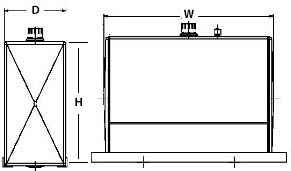# Tank Calculations

## Cylinder Tanks

Diameter(D): Outside Diameter
Length(L): End to end
pi(π): 3.14159 (approx)

((π r^2)*L)/231 = Fluid GallonsDiameter :

Length:
Gallons (*estimated):
* NOTE: Actual fluid capacity will be less than volume calculated by external dimensions.

## Rectangle Shaped Tanks

Width(W): Dimension from side to side or end to end (length
Depth(D): Dimension from front to back
Height(H): Dimension from top to bottom. Add 2" for thermal expansion

(Width*Depth*Height)/231 = Fluid GallonsWidth:

Depth:

Height: (tank only)

Gallons (*estimated):
* NOTE: Actual fluid capacity will be less than volume calculated by external dimensions.

## "L" Shaped Tanks

Width(W): Dimension from side to side or end to end (length
Depth(D): Dimension from front to back
Height(H): Dimension from top to bottom. Add 2" for thermal expansion

((Width*Depth*Height)-((Depth-D1)*(Height-H1)*Width))/231 = Fluid GallonsHeight:

Width :

H1:

D1:

Width:
Gallons (estimated):
* NOTE: Actual fluid capacity will be less than volume calculated by external dimensions.